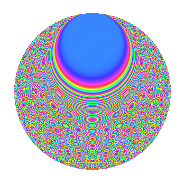# Properties

 Label 3150.2.ciLevel 3150 Weight 2 Character orbit ci Rep. character $$\chi_{3150}(407,\cdot)$$ Character field $$\Q(\zeta_{12})$$ Dimension 432 Sturm bound 1440

# Related objects

## Defining parameters

 Level: $$N$$ = $$3150 = 2 \cdot 3^{2} \cdot 5^{2} \cdot 7$$ Weight: $$k$$ = $$2$$ Character orbit: $$[\chi]$$ = 3150.ci (of order $$12$$ and degree $$4$$) Character conductor: $$\operatorname{cond}(\chi)$$ = $$45$$ Character field: $$\Q(\zeta_{12})$$ Sturm bound: $$1440$$

## Dimensions

The following table gives the dimensions of various subspaces of $$M_{2}(3150, [\chi])$$.

Total New Old
Modular forms 2976 432 2544
Cusp forms 2784 432 2352
Eisenstein series 192 0 192

## Trace form

 $$432q + 8q^{3} + O(q^{10})$$ $$432q + 8q^{3} - 48q^{11} - 8q^{12} + 216q^{16} - 8q^{18} - 16q^{21} - 24q^{23} - 16q^{27} - 8q^{33} + 16q^{36} - 48q^{37} + 72q^{38} + 192q^{41} + 96q^{47} + 16q^{48} + 176q^{51} + 32q^{57} + 8q^{63} + 88q^{66} - 24q^{67} - 16q^{72} - 32q^{78} + 240q^{81} - 96q^{82} + 120q^{83} + 72q^{86} + 40q^{87} + 96q^{91} - 24q^{92} + 32q^{93} - 72q^{97} + O(q^{100})$$

## Decomposition of $$S_{2}^{\mathrm{new}}(3150, [\chi])$$ into newform subspaces

The newforms in this space have not yet been added to the LMFDB.

## Decomposition of $$S_{2}^{\mathrm{old}}(3150, [\chi])$$ into lower level spaces

$$S_{2}^{\mathrm{old}}(3150, [\chi]) \cong$$ $$S_{2}^{\mathrm{new}}(45, [\chi])$$$$^{\oplus 8}$$$$\oplus$$$$S_{2}^{\mathrm{new}}(90, [\chi])$$$$^{\oplus 4}$$$$\oplus$$$$S_{2}^{\mathrm{new}}(225, [\chi])$$$$^{\oplus 4}$$$$\oplus$$$$S_{2}^{\mathrm{new}}(315, [\chi])$$$$^{\oplus 4}$$$$\oplus$$$$S_{2}^{\mathrm{new}}(450, [\chi])$$$$^{\oplus 2}$$$$\oplus$$$$S_{2}^{\mathrm{new}}(630, [\chi])$$$$^{\oplus 2}$$$$\oplus$$$$S_{2}^{\mathrm{new}}(1575, [\chi])$$$$^{\oplus 2}$$

## Hecke Characteristic Polynomials

There are no characteristic polynomials of Hecke operators in the database